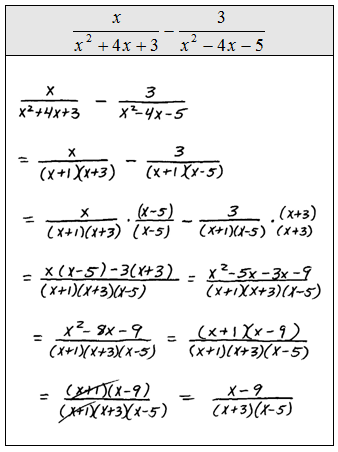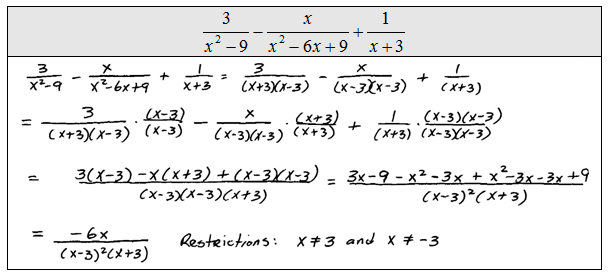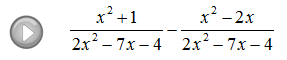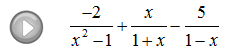## Algebra

### Adding and Subtracting Rational Expressions

Rational Expressions and Equations Playlist on YouTube

When adding or subtracting rational expressions we will need a common denominator.Simplify the resulting rational expression after adding or subtracting them.If the rational expressions that we are adding or subtracting have unlike denominators then we will need to find the equivalent fractions with the same denominator. To do this multiply both the numerator and denominator of each expression by the factors needed to obtain a common denominator. To help determine the LCD, first factor the denominators.Instructional Video: Adding and Subtracting Rational Expressions

Perform the operations and state the restrictions to the domain.For the given functions find f(x) - g(x) and state the restrictions to the domain.